Home | Mathematics | * Maxima | * Symbolic Mathematics Using Maxima 01. Acquiring, Installing and Testing 02. First Examples 03. Files and Functions 04. Creating Sets of Functions 05. Differential Equations 06. Fourier Analysis 07. A TeX Clipboard Daemon 08. Conclusion 09. TeX Clipboard Daemon Ruby ListingShare This Page
Differential Equations
P. Lutus Message Page

First Example | Second Example

(double-click any word to see its definition)

First Example

Those readers who have visited my Calculus tutorial will recognize this example — it is a common, well-understood differential equation with many real-world applications. For those who don't think about this stuff every day, a differential equation is one that expresses the relationship between a function and one or more of its derivatives. An example:

Statement

• (1) y(t) + r c y'(t) = b
• (2) y(0) = a

Let's examine the statements that describe the equation. In part (1) of the statement, we see that an unknown function y(t) is added to its derivative y'(t), which is scaled by two multiplier terms r and c.

In part (2) of the statement, an initial value is assigned to the function y(t). The meaning of this statement is that, when t = 0, y(t) = a.

Remember about differential equations that, unlike numerical equations, they describe dynamic processes — things are changing. Remember also that the derivative term y'(t) describes the rate of change in y(t).

Please think about this system for a moment. Let's say that the variable t represents time (although the equation doesn't require this interpretation). At time zero, the function y(t) equals a, therefore at that moment the derivative term y'(t) is equal to (b - a) / (r * c). Notice that y'(t), which represents the rate of change in y(t), has its largest value at time zero. Because of how the equation is written, we see that the value of y'(t) (the rate of change) becomes proportionally smaller as y(t) becomes larger. Eventually, for some very large value of t, the rate of change represented by y'(t) becomes arbitrarily small, as y(t) approaches the value of b, but never quite gets there.

Put very simply, this equation describes a system in which the rate of change in the value of y(t) depends on the remaining difference between y(t) and b, and as that difference decreases, so does the rate of change. As it happens, this equation is used to describe many natural processes, among which are:

• Electronic circuits consisting of resistors and capacitors (hence the equation's terms r and c), where the voltage on a capacitor changes in a way that depends on the current flowing through a resistor, and the value of the resistor's current depends on the voltage on the capacitor.
• Heat flow between a source of heat energy and a cooler object being heated by it (like a pot on a stove). In such a system, the temperature of the heated body changes at a rate that depends on the remaining difference in temperature between the two bodies.
• The rate of gas flow between two pressure vessels with a constricted passage between them. In this system also, the rate of gas flow depends on the remaining pressure difference, and the pressure difference declines over time.

This is by no means a comprehensive list of this equation's applications. But the statements for a differential equation are only the beginning, and not all differential equations have analytical solutions (solutions expressible as a practical function, one consisting of normal mathematical operations). Others require numerical methods and are only soluble in an approximate sense.

Let's see if Maxima can find a solution for this equation. Here are the steps:

eq:y(t)+r*c*'diff(y(t),t)=b; (equivalent to statement (1) above)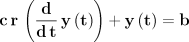atvalue(y(t),t=0,a); (equivalent to statement (2) above)
sol:desolve(eq,y(t)); ("desolve()" is one of Maxima's differential equation solvers)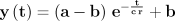(solved. "e" = base of natural logarithms)

f(t,r,c,a,b) := ev(rhs(sol)); (assign the solution to a function)Okay, we now have a function that embodies the solution to our differential equation. We can use it to solve real-world problems. Here's an example from a field in which I have spent a lot of time — electronics.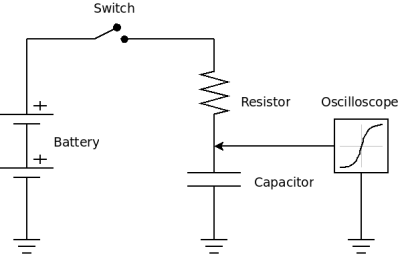R-C circuit diagram

In this experiment, we have an electronic circuit consisting of a resistor and a capacitor. At time zero, we close a switch that connects our circuit to a battery, we then use an oscilloscope to measure the voltage on the capacitor over time (see diagram this page).

Here are the Maxima instructions to set up and graph the response of the described circuit:

r:10000; (10,000 Ω)
c:100e-6; (100 µf)
a:0; (ground voltage = 0)
b:12; (battery voltage = 12)
wxplot2d(f(t,r,c,a,b),[t,0,5], [gnuplot_preamble, "set grid;"], [nticks,12]);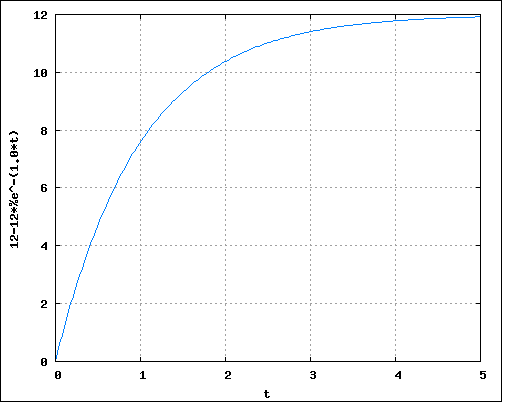Second Example

Okay, now let's move to a somewhat more complex differential equation that belongs in the same general class. In this equation, instead of a one-time event like throwing a switch that connects a circuit to a battery, we have a continuous waveform driving a system that could be an R-C circuit, or any natural system in which there is a path of resistance to the flow of something cyclical. I originally wrote this equation as part of a project to analyze the behavior of tides in channels that connect inland bays to the open ocean, but that is only one of many applications. Here again is a statement and a solution:

Statement

• (1) y(t) + r c y'(t) = m sin(ω t)

This equation has only one term, and no initial value. Let's submit this expression to Maxima and see what it comes up with:

eq:y(t)=-r*c*'diff(y(t),t)+m*sin(ω*t);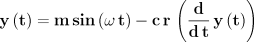sol:desolve(eq,y(t));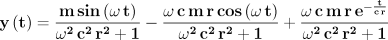Okay, I see a problem with this solution — the group of terms at the right includes the familiar "e-t/rc" expression that appears in equations with defined initial values. But, because this equation describes a continuous process with no beginning and no end, we need to set the conditions at time zero in such a way that all times will be treated equally (and the right-hand subexpression will be eliminated).

After mulling this over, I decided the correct way to characterize the initial conditions would be to submit the right-hand expression as a negative value at time zero, which has the effect of preventing the assignment of a special time-zero value. Here is that entry and the outcome:

init_val:-(c*m*r*(%e^-(t/r*c))*%omega)/(c^2*r^2*%omega^2+1);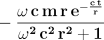atvalue(y(t),t=0,init_val); (a rather exotic initial value)
sol:desolve(eq,y(t));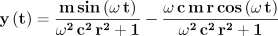(now this looks right)

f(t,r,c,ω,m) := ev(rhs(sol),fullratsimp,factor); (simplify, factor and declare a function)R-C circuit diagram with sinewave generator

Okay, we now have a working embodiment of this equation, and it turns out this form has as many real-world applications as the earlier example. Here is another electronic example using an R-C circuit, but this time with a sinewave generator driving our circuit:

m:1;
f:440; (Hertz)
r:1000; (Ω)
c:0.05e-6; (µf)
ω:2*%pi*f;

wxplot2d([sin(ω*t),f(t,r,c,ω,m)],[t,0,.005],[gnuplot_preamble, "unset key;set title 'f = 440 Hz, r = 1000, c = 100 uf'"]);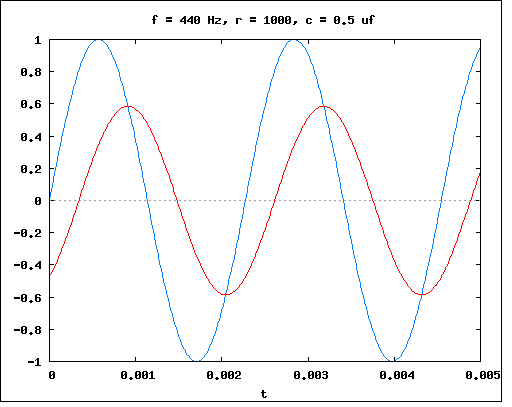-Signal Generator-  -R-C junction-

 Home | Mathematics | * Maxima | * Symbolic Mathematics Using Maxima 01. Acquiring, Installing and Testing 02. First Examples 03. Files and Functions 04. Creating Sets of Functions 05. Differential Equations 06. Fourier Analysis 07. A TeX Clipboard Daemon 08. Conclusion 09. TeX Clipboard Daemon Ruby ListingShare This Page